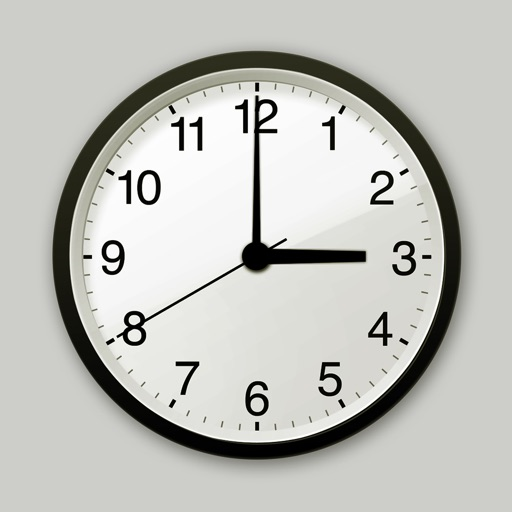Place Value
Story Problems
Geometry
Money & Time
100

10 + 10 =

20

100

Count by tens to 100.

10, 20, 30, 40, 50, 60, 70, 80, 90, 100

100

6 eyes. How many cats?

3

100

How many sides does a circle have?

0

100

How much is a penny worth?

1 cent
200

7 + 3 =

10

200

How many tens are in 15?

1

200

There are 15 cows in the field and 10 cows in the barn. How many cows in all?

25

200

What shape are the faces on a cube?

Square

200

How many hours are in a day?

24

300

8 - 4 =

4

300

How many ones are in 24?

4

300

10 frogs on a log. 6 jump into the pond. How many are left on the log?

4

300

How many vertices does a cone have?

1

300

How much is a quarter worth?

25 cents

400

50 + 50 =

100

400

What is 2 tens plus 3 tens equal?

50

400

12 legs. How many sheep?

3
400

How many triangles do you need to make a hexagon?

6

400

How many dimes do you need to make a dollar?

10

500

23 - 17 =

6
500

How many tens are in 100?

10

500

Henry has one dollar. He buys a stamp for 55 cents. How much money does he have left?

45 cents

500

How many vertices does a cube have?

8

500What time does the clock show?

3:00

Click to zoom